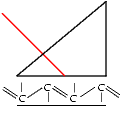# RSim     Fresnel formulas

Here we deal with the reflection of bulk material (fresnel formulas)
To obtain the classical fresnel plot choose n0= 1.0 (air) and n7= 1.5151 ( glass BK7). You can study the different spectra for s and p polarized light and measure the brewster angle. In the example: s polarization = black curve, p polarization = red curve